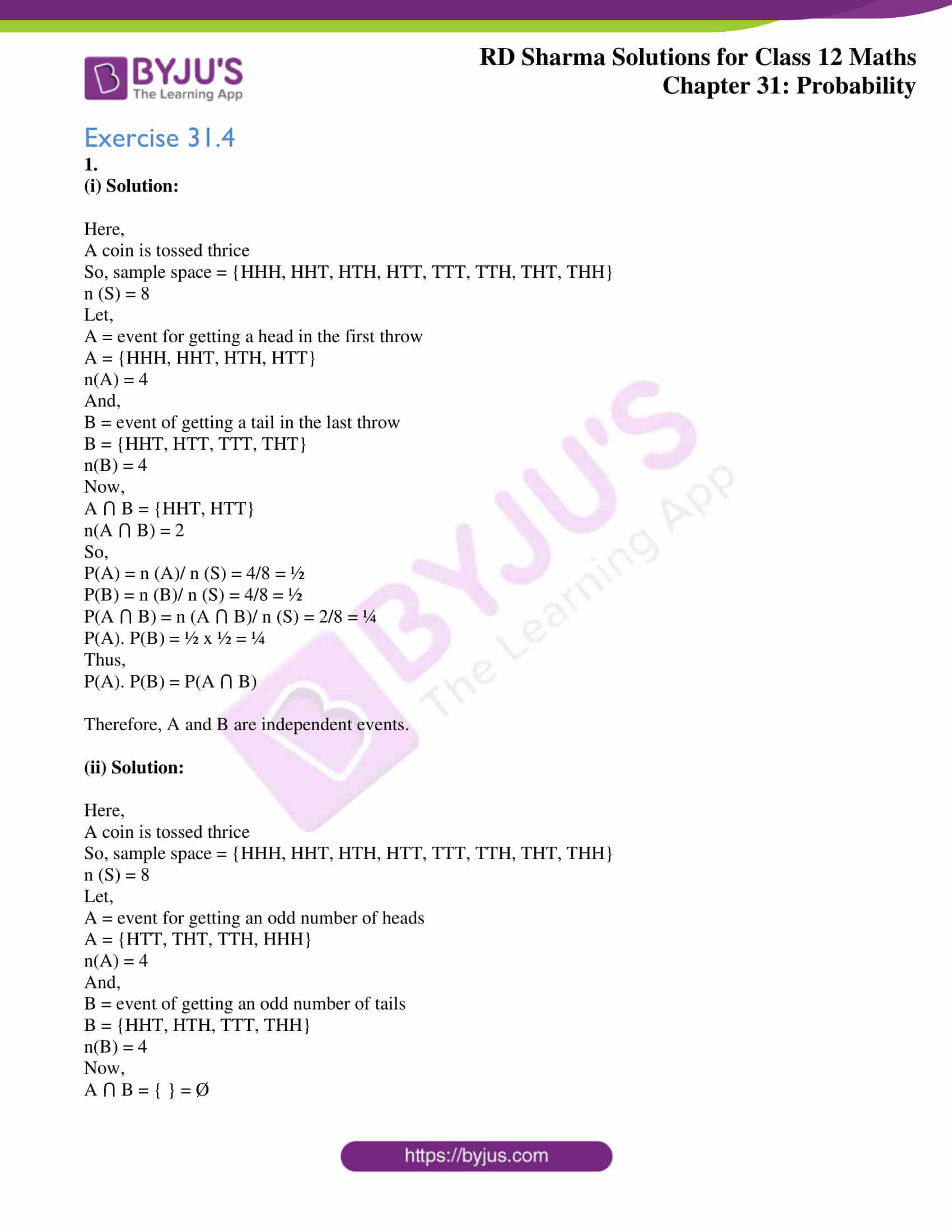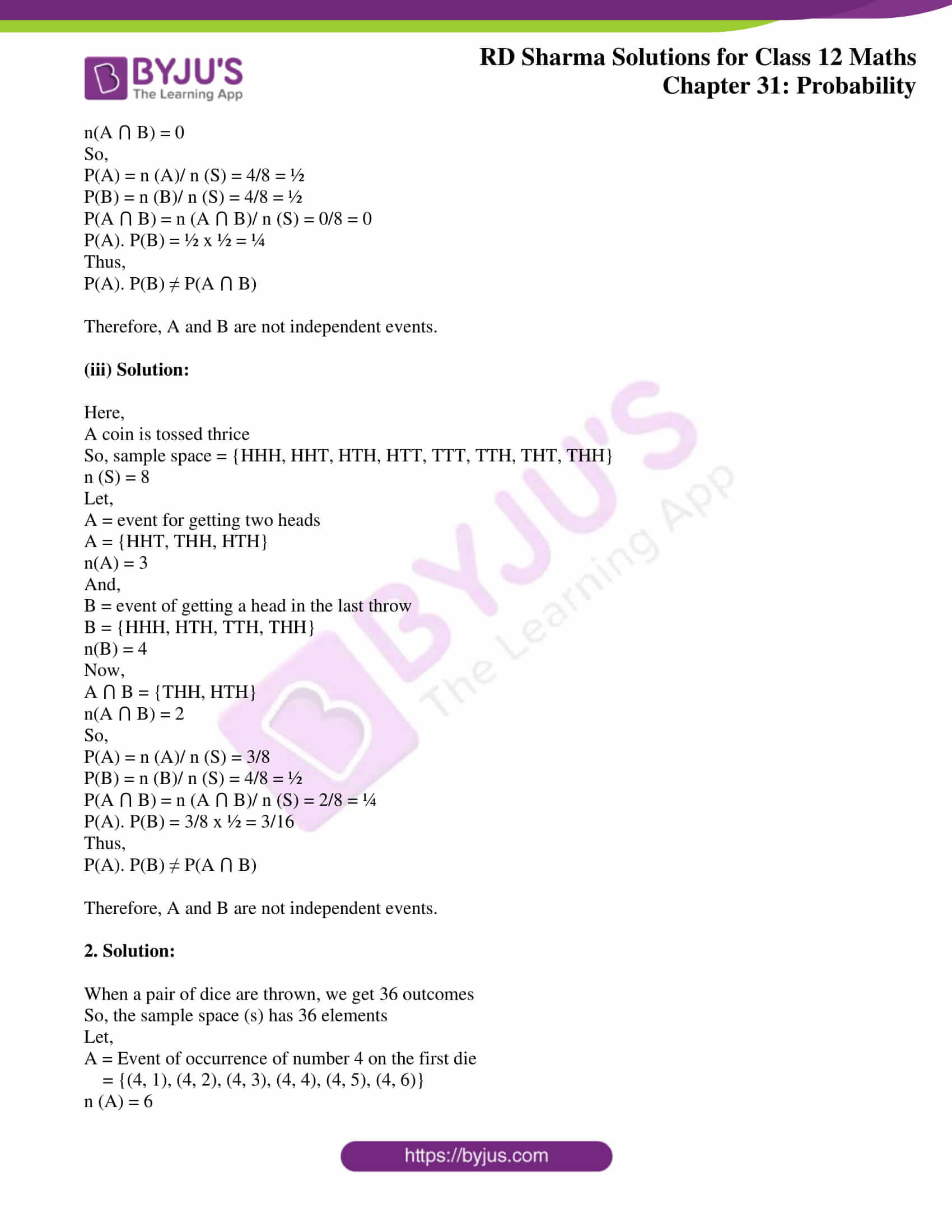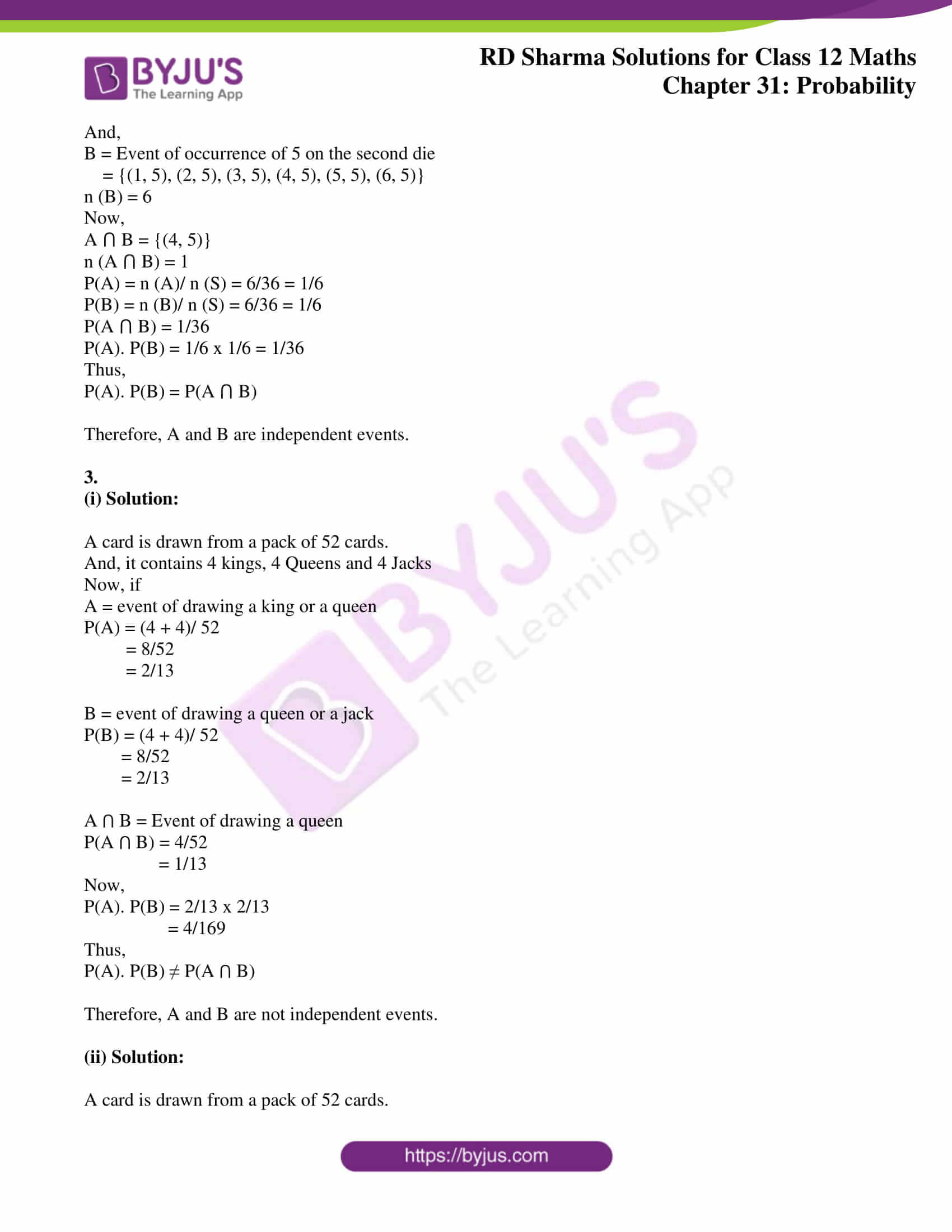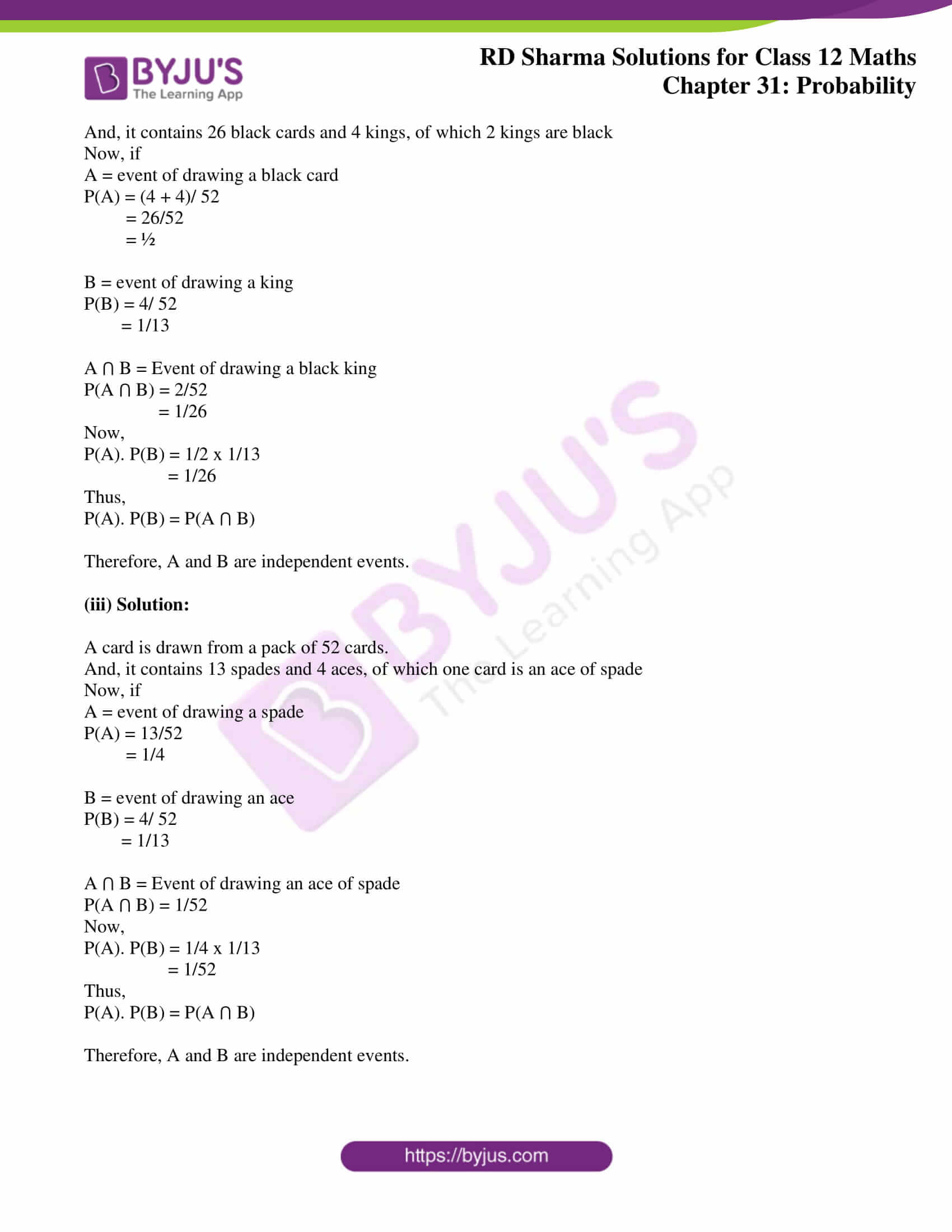# RD Sharma Solutions Class 12 Probability Exercise 31.4

RD Sharma Solutions for Class 12 Maths Chapter 31 Probability Exercise 31.4 can be found here. Students who aspire to secure high scores in their Maths examinations can refer to RD Sharma Solutions. By far, this is the best guide to learn solving methods and improve problem-solving skills. Practising the RD Sharma Class 12 Solutions regularly will help attain a firm grip over the subject. Further, the PDF containing the solutions on this exercise is available for free and can be downloaded from the link given below.

## Download PDF of RD Sharma Solutions for Class 12 Maths Chapter 31 Exercise 4### Access RD Sharma Solutions for Class 12 Maths Chapter 31 Exercise 4

Exercise 31.4

1.

(i) Solution:

Here,

A coin is tossed thrice

So, sample space = {HHH, HHT, HTH, HTT, TTT, TTH, THT, THH}

n (S) = 8

Let,

A = event for getting a head in the first throw

A = {HHH, HHT, HTH, HTT}

n(A) = 4

And,

B = event of getting a tail in the last throw

B = {HHT, HTT, TTT, THT}

n(B) = 4

Now,

A ⋂ B = {HHT, HTT}

n(A ⋂ B) = 2

So,

P(A) = n (A)/ n (S) = 4/8 = ½

P(B) = n (B)/ n (S) = 4/8 = ½

P(A ⋂ B) = n (A ⋂ B)/ n (S) = 2/8 = ¼

P(A). P(B) = ½ x ½ = ¼

Thus,

P(A). P(B) = P(A ⋂ B)

Therefore, A and B are independent events.

(ii) Solution:

Here,

A coin is tossed thrice

So, sample space = {HHH, HHT, HTH, HTT, TTT, TTH, THT, THH}

n (S) = 8

Let,

A = event for getting an odd number of heads

A = {HTT, THT, TTH, HHH}

n(A) = 4

And,

B = event of getting an odd number of tails

B = {HHT, HTH, TTT, THH}

n(B) = 4

Now,

A ⋂ B = { } = Ø

n(A ⋂ B) = 0

So,

P(A) = n (A)/ n (S) = 4/8 = ½

P(B) = n (B)/ n (S) = 4/8 = ½

P(A ⋂ B) = n (A ⋂ B)/ n (S) = 0/8 = 0

P(A). P(B) = ½ x ½ = ¼

Thus,

P(A). P(B) ≠ P(A ⋂ B)

Therefore, A and B are not independent events.

(iii) Solution:

Here,

A coin is tossed thrice

So, sample space = {HHH, HHT, HTH, HTT, TTT, TTH, THT, THH}

n (S) = 8

Let,

A = event for getting two heads

A = {HHT, THH, HTH}

n(A) = 3

And,

B = event of getting a head in the last throw

B = {HHH, HTH, TTH, THH}

n(B) = 4

Now,

A ⋂ B = {THH, HTH}

n(A ⋂ B) = 2

So,

P(A) = n (A)/ n (S) = 3/8

P(B) = n (B)/ n (S) = 4/8 = ½

P(A ⋂ B) = n (A ⋂ B)/ n (S) = 2/8 = ¼

P(A). P(B) = 3/8 x ½ = 3/16

Thus,

P(A). P(B) ≠ P(A ⋂ B)

Therefore, A and B are not independent events.

2. Solution:

When a pair of dice are thrown, we get 36 outcomes

So, the sample space (s) has 36 elements

Let,

A = Event of occurrence of number 4 on the first die

= {(4, 1), (4, 2), (4, 3), (4, 4), (4, 5), (4, 6)}

n (A) = 6

And,

B = Event of occurrence of 5 on the second die

= {(1, 5), (2, 5), (3, 5), (4, 5), (5, 5), (6, 5)}

n (B) = 6

Now,

A ⋂ B = {(4, 5)}

n (A ⋂ B) = 1

P(A) = n (A)/ n (S) = 6/36 = 1/6

P(B) = n (B)/ n (S) = 6/36 = 1/6

P(A ⋂ B) = 1/36

P(A). P(B) = 1/6 x 1/6 = 1/36

Thus,

P(A). P(B) = P(A ⋂ B)

Therefore, A and B are independent events.

3.

(i) Solution:

A card is drawn from a pack of 52 cards.

And, it contains 4 kings, 4 Queens and 4 Jacks

Now, if

A = event of drawing a king or a queen

P(A) = (4 + 4)/ 52

= 8/52

= 2/13

B = event of drawing a queen or a jack

P(B) = (4 + 4)/ 52

= 8/52

= 2/13

A ⋂ B = Event of drawing a queen

P(A ⋂ B) = 4/52

= 1/13

Now,

P(A). P(B) = 2/13 x 2/13

= 4/169

Thus,

P(A). P(B) ≠ P(A ⋂ B)

Therefore, A and B are not independent events.

(ii) Solution:

A card is drawn from a pack of 52 cards.

And, it contains 26 black cards and 4 kings, of which 2 kings are black

Now, if

A = event of drawing a black card

P(A) = (4 + 4)/ 52

= 26/52

= ½

B = event of drawing a king

P(B) = 4/ 52

= 1/13

A ⋂ B = Event of drawing a black king

P(A ⋂ B) = 2/52

= 1/26

Now,

P(A). P(B) = 1/2 x 1/13

= 1/26

Thus,

P(A). P(B) = P(A ⋂ B)

Therefore, A and B are independent events.

(iii) Solution:

A card is drawn from a pack of 52 cards.

And, it contains 13 spades and 4 aces, of which one card is an ace of spade

Now, if

A = event of drawing a spade

P(A) = 13/52

= 1/4

B = event of drawing an ace

P(B) = 4/ 52

= 1/13

A ⋂ B = Event of drawing an ace of spade

P(A ⋂ B) = 1/52

Now,

P(A). P(B) = 1/4 x 1/13

= 1/52

Thus,

P(A). P(B) = P(A ⋂ B)

Therefore, A and B are independent events.